Concepts

Class 9
Chapter 8 Class 9 - Motion

## What is Average Speed?

It is the total distance travelled divided by total time taken.

Average Speed = Total Distance/Total Time Taken

#### Calculating Average Speed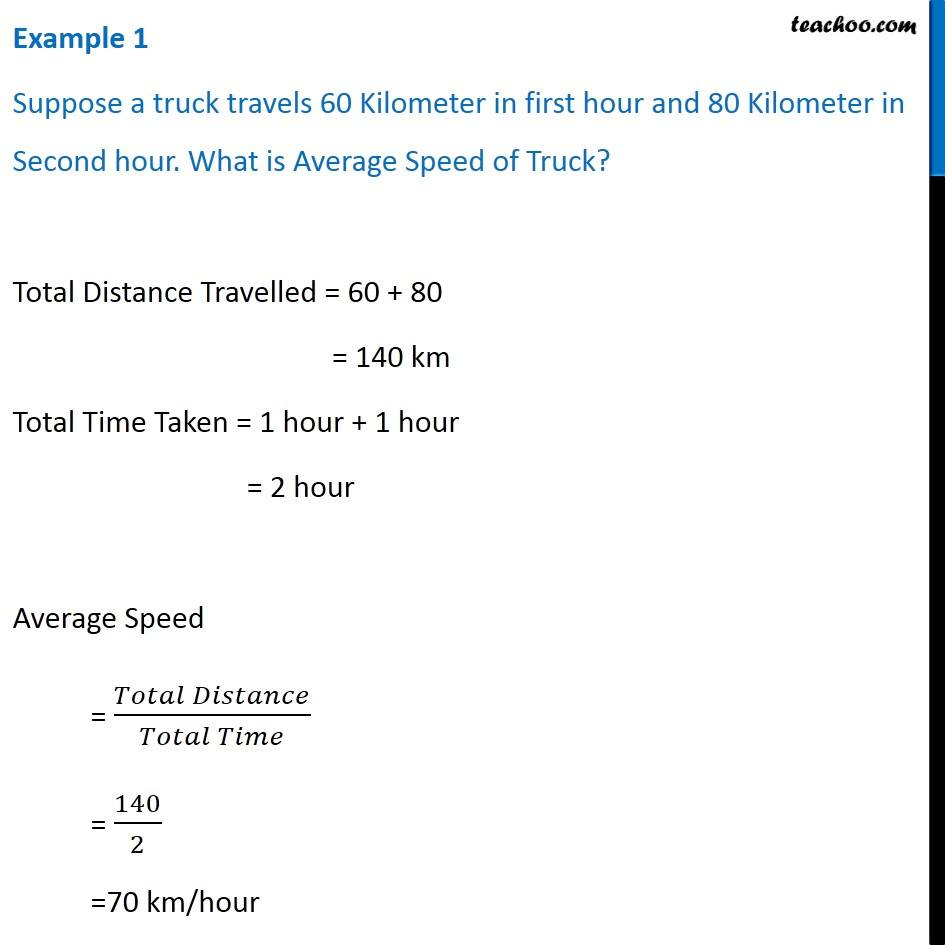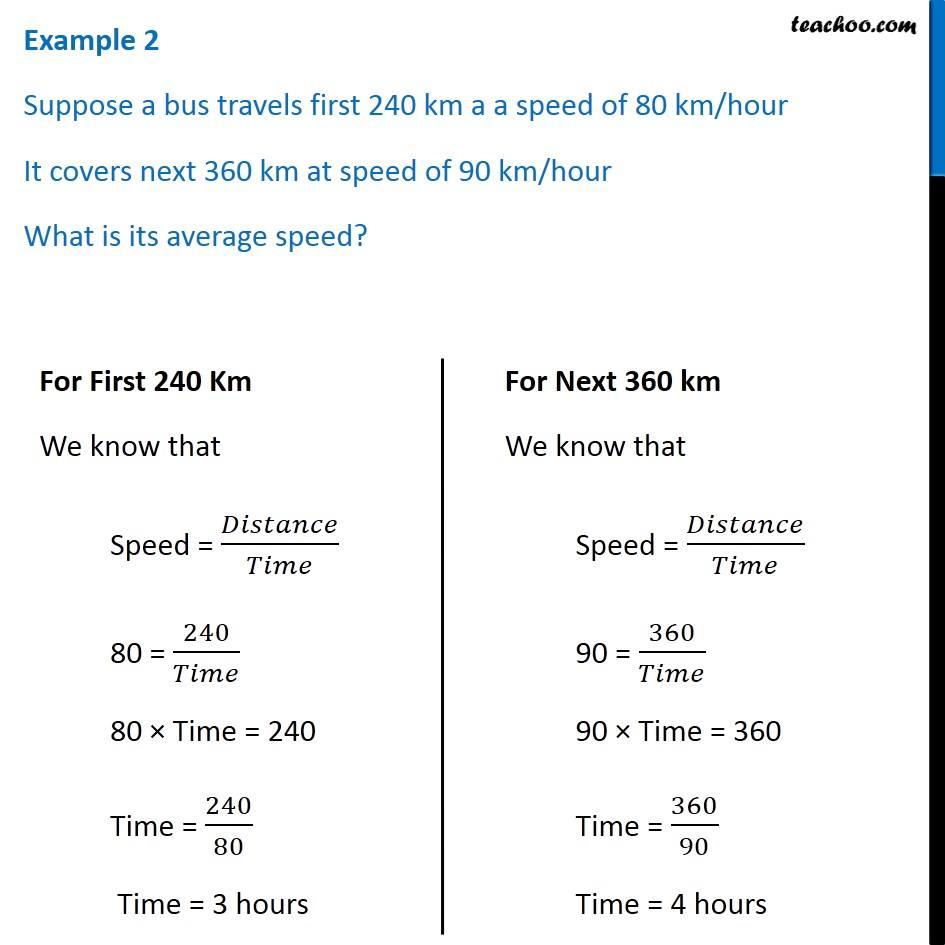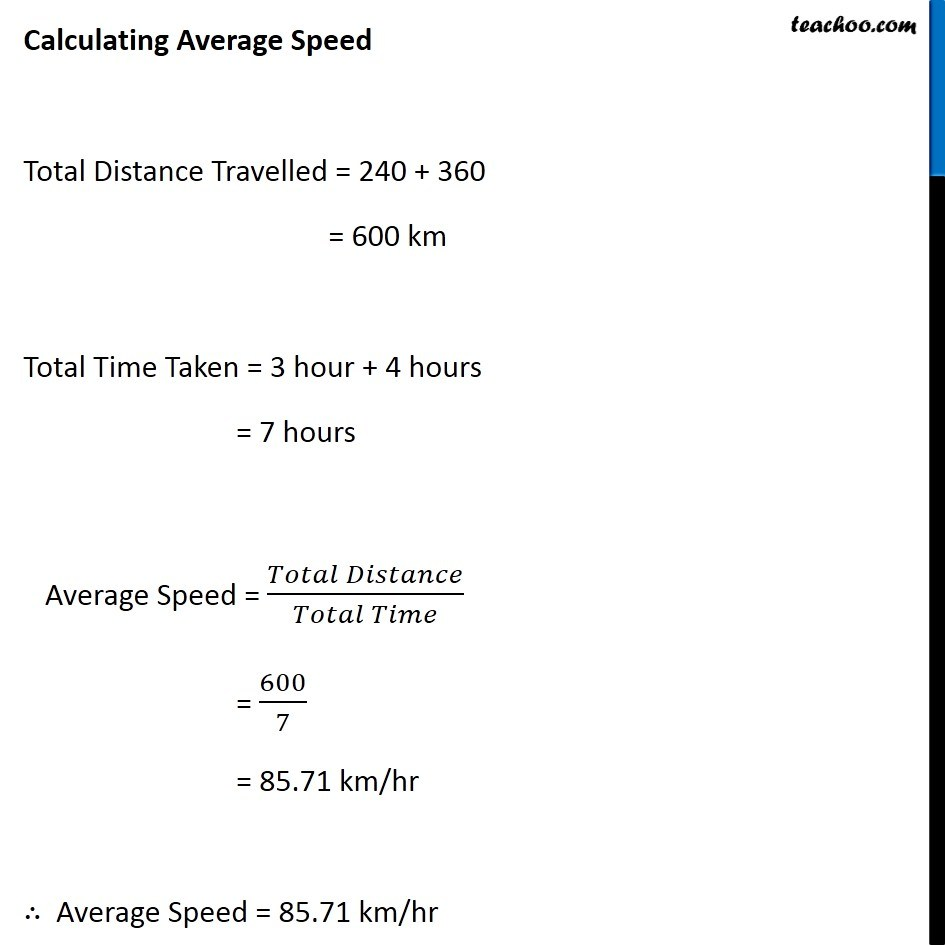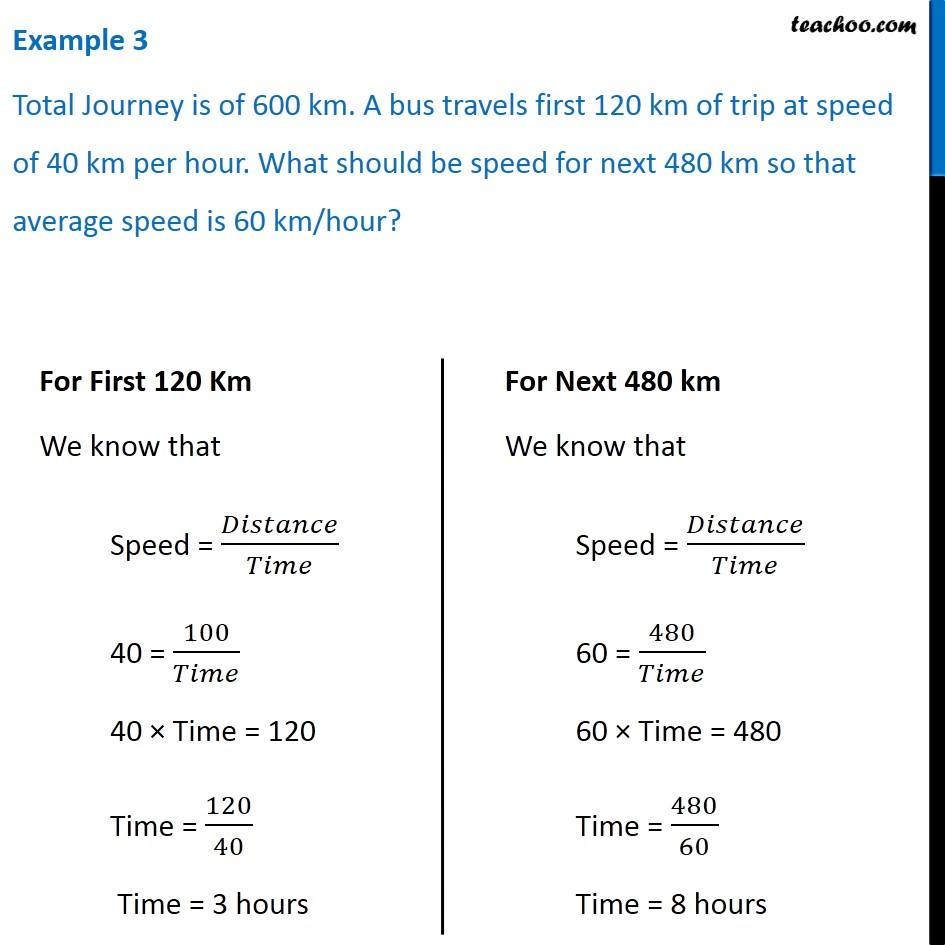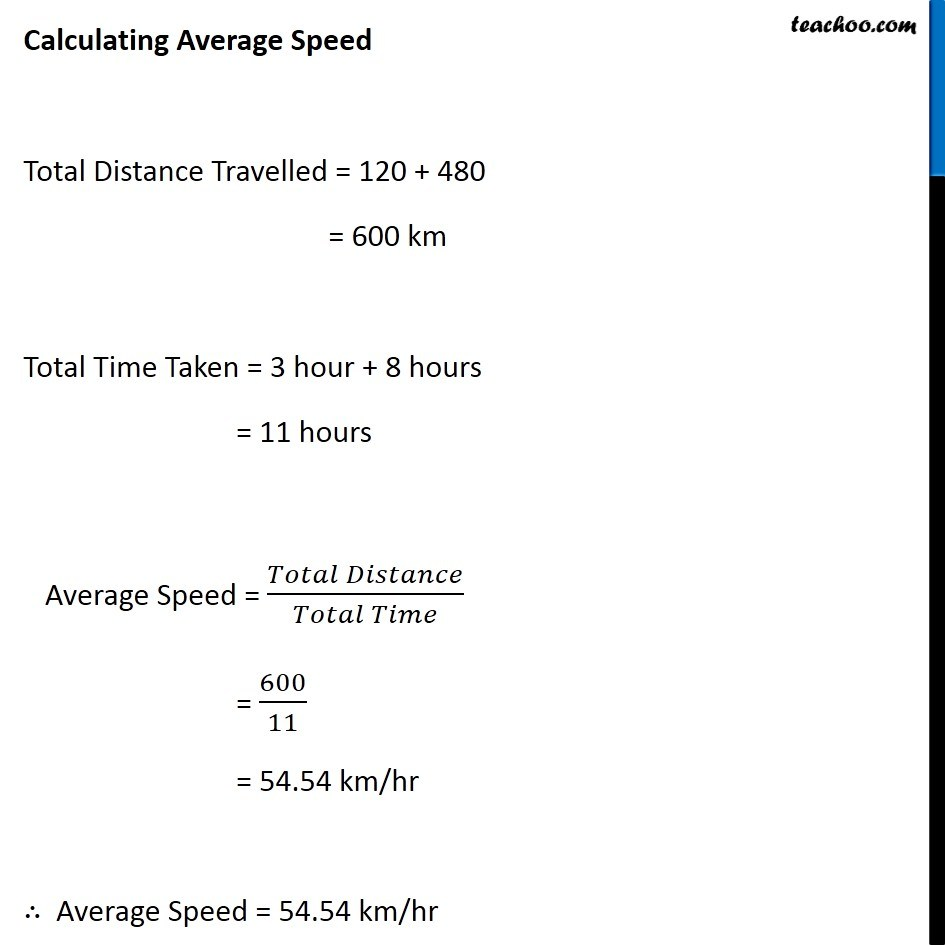## Questions

NCERT Question 3 - Abdul, while driving to school, computes the average speed for his trip to be 20 km h–1. On his return trip along the same route, there is less traffic and the average speed is 30 km h–1. What is the average speed for Abdul’s trip?

NCERT Question 10 - An artificial satellite is moving in a circular orbit of radius 42250 km. Calculate its speed if it takes 24 hours to revolve around the earth

Example 8.1 - An object travels 16 m in 4 s and then another 16 m in 2 s. What is the average speed of the object?

Example 8.2 - The Odometer of a car reads 2000 km at the start of a trip and 2400 km at the end of the trip. If the trip took 8 h, calculate the average speed of the car in km h −1 and m s -1 .

Learn in your speed, with individual attention - Teachoo Maths 1-on-1 Class

### Transcript

Example 1 Suppose a truck travels 60 Kilometer in first hour and 80 Kilometer in Second hour. What is Average Speed of Truck? Total Distance Travelled = 60 + 80 = 140 km Total Time Taken = 1 hour + 1 hour = 2 hour Average Speed = (𝑇𝑜𝑡𝑎𝑙 𝐷𝑖𝑠𝑡𝑎𝑛𝑐𝑒)/(𝑇𝑜𝑡𝑎𝑙 𝑇𝑖𝑚𝑒) = 140/2 =70 km/hour Example 2 Suppose a bus travels first 240 km a a speed of 80 km/hour It covers next 360 km at speed of 90 km/hour What is its average speed? For First 240 Km We know that Speed = 𝐷𝑖𝑠𝑡𝑎𝑛𝑐𝑒/𝑇𝑖𝑚𝑒 80 = 240/𝑇𝑖𝑚𝑒 80 × Time = 240 Time = 240/80 Time = 3 hours For Next 360 km We know that Speed = 𝐷𝑖𝑠𝑡𝑎𝑛𝑐𝑒/𝑇𝑖𝑚𝑒 90 = 360/𝑇𝑖𝑚𝑒 90 × Time = 360 Time = 360/90 Time = 4 hours Calculating Average Speed Total Distance Travelled = 240 + 360 = 600 km Total Time Taken = 3 hour + 4 hours = 7 hours Average Speed = (𝑇𝑜𝑡𝑎𝑙 𝐷𝑖𝑠𝑡𝑎𝑛𝑐𝑒)/(𝑇𝑜𝑡𝑎𝑙 𝑇𝑖𝑚𝑒) = 600/7 = 85.71 km/hr ∴ Average Speed = 85.71 km/hr Example 3 Total Journey is of 600 km. A bus travels first 120 km of trip at speed of 40 km per hour. What should be speed for next 480 km so that average speed is 60 km/hour? For First 120 Km We know that Speed = 𝐷𝑖𝑠𝑡𝑎𝑛𝑐𝑒/𝑇𝑖𝑚𝑒 40 = 100/𝑇𝑖𝑚𝑒 40 × Time = 120 Time = 120/40 Time = 3 hours For Next 480 km We know that Speed = 𝐷𝑖𝑠𝑡𝑎𝑛𝑐𝑒/𝑇𝑖𝑚𝑒 60 = 480/𝑇𝑖𝑚𝑒 60 × Time = 480 Time = 480/60 Time = 8 hours Calculating Average Speed Total Distance Travelled = 120 + 480 = 600 km Total Time Taken = 3 hour + 8 hours = 11 hours Average Speed = (𝑇𝑜𝑡𝑎𝑙 𝐷𝑖𝑠𝑡𝑎𝑛𝑐𝑒)/(𝑇𝑜𝑡𝑎𝑙 𝑇𝑖𝑚𝑒) = 600/11 = 54.54 km/hr ∴ Average Speed = 54.54 km/hr